# Regularisers¶

Torchbearer has a number of built-in regularisers which can be added to any image problem with a simple callback. In the example we will quickly demonstrate each one and give an example of how they modify the image.

Note: The easiest way to use this tutorial is as a colab notebook, which allows you to dive in with no setup. We recommend you enable a free GPU with

Runtime   →   Change runtime type   →   Hardware Accelerator: GPU

## Install Torchbearer¶

First we install torchbearer if needed.

In :
try:
import torchbearer
except:
!pip install -q torchbearer
import torchbearer

print(torchbearer.__version__)

0.5.1.dev


## Data¶

For simplicity and speed, this example will use MNIST. MNIST also has the advantage that it is usually quite easy to overfit on, and so if you want to run this example with a more powerful model and for a few more epochs then you should see be able to see the power of each regulariser.

In :
import torch
from torchvision import datasets, transforms
from torchbearer.cv_utils import DatasetValidationSplitter

transform = transforms.Compose([
transforms.ToTensor(),
transforms.Normalize((0.1307,), (0.3081,))
])
BATCH_SIZE = 128

splitter = DatasetValidationSplitter(len(dataset), 0.1)
trainset = splitter.get_train_dataset(dataset)
valset = splitter.get_val_dataset(dataset)

traingen = torch.utils.data.DataLoader(trainset, pin_memory=True, batch_size=BATCH_SIZE, shuffle=True, num_workers=10)
valgen = torch.utils.data.DataLoader(valset, pin_memory=True, batch_size=BATCH_SIZE, shuffle=True, num_workers=10)
testgen = torch.utils.data.DataLoader(testset, pin_memory=True, batch_size=BATCH_SIZE, shuffle=False, num_workers=10)


## Model¶

We take the same model as the quickstart example and modify it to run on MNIST. This should run very quickly which will help us see the impact of the reguliarisers.

In :
import torch.nn as nn

class SimpleModel(nn.Module):
def __init__(self):
super(SimpleModel, self).__init__()
self.convs = nn.Sequential(
nn.Conv2d(1, 16, stride=2, kernel_size=3),
nn.BatchNorm2d(16),
nn.ReLU(),
nn.Conv2d(16, 32, stride=2, kernel_size=3),
nn.BatchNorm2d(32),
nn.ReLU(),
nn.Conv2d(32, 64, stride=2, kernel_size=3),
nn.BatchNorm2d(64),
nn.ReLU()
)

self.classifier = nn.Linear(64*2*2, 10)

def forward(self, x):
x = self.convs(x)
x = x.view(-1, 64*2*2)
return self.classifier(x)

model = SimpleModel()


## Set of Regularisers¶

Torchbearer has the following built-in reguliarisers:

• Cutout: Randomly replaces an area of the image with a constant value
• RandomErase: Randomly replaces an area of the image with noise
• Sample Pairing: Averages two images without change to targets
• MixUp: Linearly combines two images and their labels
• CutMix: Randomly replaces a region of an image with a region of another. Replaces targets based on the percentage of each image
• Label Smoothing: Smooths the labels according to an epsilon, resulting in them being float values

Here we create the callbacks for each of these in turn.

In :
from torchbearer.callbacks import Cutout, RandomErase, Mixup, SamplePairing, LabelSmoothingRegularisation, CutMix, BCPlus

cutout = Cutout(n_holes=1, length=8, constant=1)
random_erase = RandomErase(n_holes=2, length=6)
mixup = Mixup()
smoothing = LabelSmoothingRegularisation(0.1, 10)
cutmix = CutMix(1., 10)
bcplus = BCPlus(classes=10)

# Do sample pairing for the first two epochs for demonstration.
# We recommend using the policy from the paper (policy=None) for training purposes
pairing = SamplePairing(SamplePairing.default_policy(0, 2, 8, 2))


## Visualising¶

All of the regularisers that we are going to show are very visual. We would like to see how they modify the image so we create a MakeGrid callback form imaging to show the input data once every epoch.

In :
import torchbearer.callbacks.imaging as imag

make_grid = imag.MakeGrid(torchbearer.INPUT, num_images=8, nrow=8, transform=transforms.Normalize((-0.1307/0.3081,), (1/0.3081,)))
make_grid = make_grid.on_train().to_pyplot()


## Trial¶

Now lets create a number of trails and observe how each of the regularisers changes the results.

In :
import torch.optim as optim

device = 'cuda' if torch.cuda.is_available() else 'cpu'
loss = nn.CrossEntropyLoss()

import torchbearer
from torchbearer import Trial

### Sample Pairing
model = SimpleModel()

callbacks = [pairing, make_grid]
trial_sp = Trial(model, optimizer, loss, metrics=['loss', 'acc'], callbacks=callbacks).to(device)
trial_sp.with_generators(train_generator=traingen, val_generator=valgen, test_generator=testgen)
history_sp = trial_sp.run(epochs=5, verbose=1)

# Only sample pairing changes the regularisation based on the epoch
# From now on we hack the make_grid callback to only print once per training through the only_if decorator
from torchbearer.callbacks import only_if
make_grid.on_step_training = only_if(lambda state: state[torchbearer.EPOCH] == 0)(make_grid.on_step_training)

### Mixup
model = SimpleModel()

callbacks = [mixup, make_grid]
trial_mu = Trial(model, optimizer, Mixup.mixup_loss, metrics=['acc', 'loss'], callbacks=callbacks).to(device)
trial_mu.with_generators(train_generator=traingen, val_generator=valgen, test_generator=testgen)
history_mu = trial_mu.run(epochs=5, verbose=1)

### Cutout
model = SimpleModel()

callbacks = [cutout, make_grid]
trial_co = Trial(model, optimizer, loss, metrics=['acc', 'loss'], callbacks=callbacks).to(device)
trial_co.with_generators(train_generator=traingen, val_generator=valgen, test_generator=testgen)
history_cutout = trial_co.run(epochs=5, verbose=1)

### Random Erase
model = SimpleModel()

callbacks = [random_erase, make_grid]
trial_re = Trial(model, optimizer, loss, metrics=['acc', 'loss'], callbacks=callbacks).to(device)
trial_re.with_generators(train_generator=traingen, val_generator=valgen, test_generator=testgen)
history_erase = trial_re.run(epochs=5, verbose=1)

### CutMix
model = SimpleModel()

callbacks = [cutmix, make_grid]
trial_cm = Trial(model, optimizer, nn.BCEWithLogitsLoss(), metrics=['acc', 'loss', 'cat_acc'], callbacks=callbacks).to(device)
trial_cm.with_generators(train_generator=traingen, val_generator=valgen, test_generator=testgen)
history_cutmix = trial_cm.run(epochs=5, verbose=1)

### BCPlus
model = SimpleModel()

callbacks = [bcplus, make_grid]
trial_bc = Trial(model, optimizer, BCPlus.bc_loss, metrics=['acc', 'loss'], callbacks=callbacks).to(device)
trial_bc.with_generators(train_generator=traingen, val_generator=valgen, test_generator=testgen)
history_bcplus = trial_bc.run(epochs=5, verbose=1)

### Label Smoothing - Doesn't modify the image, so we dont show them here.
# Also add a separate catagorical accuracy metric since the default for BCE losses is binary accurcy.
model = SimpleModel()

callbacks = [smoothing]
trial_ls = Trial(model, optimizer, criterion=nn.BCEWithLogitsLoss(), metrics=['acc', 'loss', 'cat_acc'], callbacks=callbacks).to(device)
trial_ls.with_generators(train_generator=traingen, val_generator=valgen, test_generator=testgen)
history_ls = trial_ls.run(epochs=5, verbose=1)

### Baseline - no regulariser
model = SimpleModel()

callbacks = []
trial_base = Trial(model, optimizer, loss, metrics=['loss', 'acc'], callbacks=callbacks).to(device)
trial_base.with_generators(train_generator=traingen, val_generator=valgen, test_generator=testgen)
history_base = trial_base.run(epochs=5, verbose=1)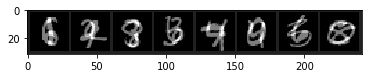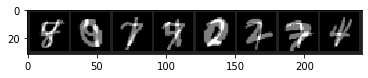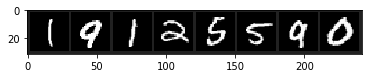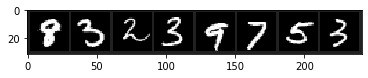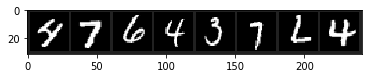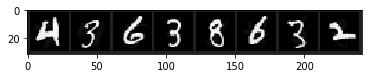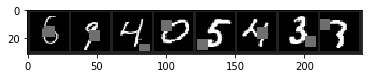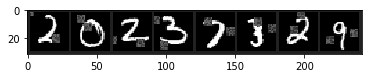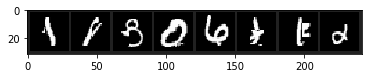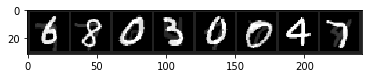## Results¶

We show some results for these models, quoting the accuracies on validation and test.

In :
print('Final val acc for baseline: {}'.format(history_base[-1]['val_acc']))

print('Final val acc for cutout: {}'.format(history_cutout[-1]['val_acc']))
print('Final val acc for random erase: {}'.format(history_erase[-1]['val_acc']))
print('Final val acc for mixup: {}'.format(history_mu[-1]['val_mixup_acc']))
print('Final val acc for cutmix: {}'.format(history_cutmix[-1]['val_acc']))
print('Final val acc for BC+: {}'.format(history_bcplus[-1]['val_acc']))
print('Final val acc for sample pairing: {}'.format(history_sp[-1]['val_acc']))
print('Final val acc for label smoothing: {}'.format(history_ls[-1]['val_acc']))

print('\n')
print('Final test acc for baseline: {}'.format(trial_base.evaluate(verbose=0, data_key=torchbearer.TEST_DATA)['test_acc']))
print('Final test acc for cutout: {}'.format(trial_co.evaluate(verbose=0, data_key=torchbearer.TEST_DATA)['test_acc']))
print('Final test acc for random erase: {}'.format(trial_re.evaluate(verbose=0, data_key=torchbearer.TEST_DATA)['test_acc']))
print('Final test acc for mixup: {}'.format(trial_mu.evaluate(verbose=0, data_key=torchbearer.TEST_DATA)['test_mixup_acc']))
print('Final test acc for cutmix: {}'.format(trial_cm.evaluate(verbose=0, data_key=torchbearer.TEST_DATA)['test_acc']))
print('Final test acc for BC+: {}'.format(trial_bc.evaluate(verbose=0, data_key=torchbearer.TEST_DATA)['test_acc']))
print('Final test acc for sample pairing: {}'.format(trial_sp.evaluate(verbose=0, data_key=torchbearer.TEST_DATA)['test_acc']))
print('Final test acc for label smoothing: {}'.format(trial_ls.evaluate(verbose=0, data_key=torchbearer.TEST_DATA)['test_acc']))

Final val acc for baseline: 0.9858333468437195
Final val acc for cutout: 0.9864999651908875
Final val acc for random erase: 0.9868333339691162
Final val acc for mixup: 0.9803333282470703
Final val acc for cutmix: 0.9736666679382324
Final val acc for BC+: 0.9706666469573975
Final val acc for sample pairing: 0.984666645526886
Final val acc for label smoothing: 0.9868333339691162

Final test acc for baseline: 0.9860000014305115
Final test acc for cutout: 0.9876999855041504
Final test acc for random erase: 0.9876999855041504
Final test acc for mixup: 0.9829999804496765
Final test acc for cutmix: 0.9747999906539917
Final test acc for BC+: 0.9770999550819397
Final test acc for sample pairing: 0.9865999817848206
Final test acc for label smoothing: 0.9869999885559082


We now plot the validation accuracies over time. In reality it would be better to log to tensorboard, visdom or live loss plot, but for this example we just use pyplot. From this small amount of training we cannot draw many conclusions, we shouldn't expect to see much difference between the regularised and baseline models. If, however, we were to run these models for longer we would hope to see them out perform the baseline when the baseline starts to overfit.

In :
import matplotlib.pyplot as plt

cutout_accs = [history_cutout[i]['val_acc'] for i in range(len(history_cutout))]
erase_accs = [history_erase[i]['val_acc'] for i in range(len(history_erase))]
mixup_accs = [history_mu[i]['val_mixup_acc'] for i in range(len(history_mu))]
pairing_accs = [history_sp[i]['val_acc'] for i in range(len(history_sp))]
cutmix_accs = [history_cutmix[i]['val_acc'] for i in range(len(history_cutmix))]
bcplus_accs = [history_bcplus[i]['val_acc'] for i in range(len(history_bcplus))]
smoothing_accs = [history_ls[i]['val_acc'] for i in range(len(history_ls))]
baseline_accs = [history_base[i]['val_acc'] for i in range(len(history_base))]

plt.plot(cutout_accs, label='cutout')
plt.plot(erase_accs, label='erase')
plt.plot(mixup_accs, label='mixup')
plt.plot(pairing_accs, label='pairing')
plt.plot(cutmix_accs, label='cutmix')
plt.plot(bcplus_accs, label='bc+')
plt.plot(smoothing_accs, label='smoothing')
plt.plot(baseline_accs, label='baseline')
plt.legend()
plt.ylabel('Validation Accuracy')
plt.xlabel('Epoch')
plt.show()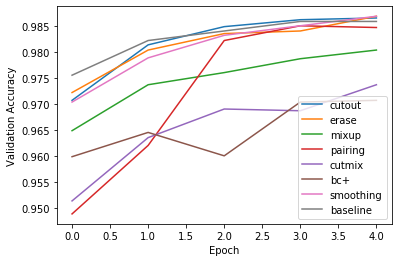In [ ]: